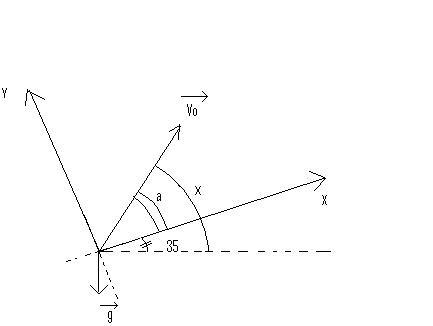# Maximum range equation

## Homework Statement

A projectile is launched 80m/s into the air at angle x to the horizontal off the top edge of an infinitely long hill(inclined plane). The hill makes a 35 degrees angle to the horizontal
a) Derive an equation for the range(maximum horizontal displacement).
b) At what angle will the projectile have maximum range?

## Homework Equations

I know the equation for range of an even surface and how to derive it but I don't think that will be of help here.

## The Attempt at a Solution

Is this question even possible?
I'm tried to derive an equation but with no luck. That's the hard part. I think I just need to differentiate the equation to get the angle of maximum range.

LowlyPion
Homework Helper
You can start with the usual equations and the components of the initial velocity in θ.

The slope adds the additional relationship between the x and y as in

y/x = tan35

U have several way to approach this question.

1)U may establish the equation representing the way the projectile moving. Establish the equation of the hill. Find the cross point.

2)(I recommend) U consider the line representing the hill the x axis(picture). Do some math and find the result.Consider the projectile moves along x and y axis seperately:

x axis: x=Vo.cosa.t - (sin35.g.t^2)/2 (1)
y axis: y=Vo.sina.t - (cos35.g.t^2)/2 (2)

When the projectile touch the hill, y = 0
So t=0 (starting point) or t= 2.Vo.sina/(cos35.g) (the time it falls)

Replace t= 2.Vo.sina/(cos35.g) in the equation (1), and u will find the range along the hill (pretty complicated).

To convert the range along the hill to the horizontal range, just multiply the x with cos35.

Now u get the result, try to find at which angle the range is max.

Hard question it is, but do it carefully and u can solve it. Be happy :D